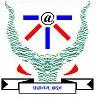Alex and the Substring (Med)
Tag(s):

## Easy-Med, Easy-Medium

Problem
Editorial
Analytics

Alex used to be fond of numbers for long but now the thing that amuses her the most are Strings. So, she built an array (A) consisting of numbers and a string (S) of lowercase English characters and mapped each element of the array with string such that

A0 is mapped to S0 ,

A1 is mapped to S1 and so on...

At that time Betty, a friend of Alex came and asked her to find the number of substrings [i, j] ,where 'i' is strictly less than j , such that S[i] = S[j] and the sum of the array elements in the range [i+1,j-1] is equal to zero.

Note: If S[i] = S[j] for some (i + 1 = j) , the sum of array elements between them will be considered as 0.

$Input :$

- The first line of the input contains an integer T denoting the number of test cases.

- The first line of each test case contains a single integer n, the length of the string (or the array).

- The second line contains the string S.

- The third line contains n space separated integers denoting A0 to An-1

$Output :$

Print one integer, denoting the answer.

$Constraints :$

1<=T<=10

1<=n<=100000

(-100000<=Ai<=100000)

Problem setter: Tanya

SAMPLE INPUT
1
5
aabca
1 1 -1 1 1
SAMPLE OUTPUT
2
Time Limit: 1.0 sec(s) for each input file.
Memory Limit: 256 MB
Source Limit: 1024 KB
Marking Scheme: Marks are awarded when all the testcases pass.
Allowed Languages: Bash, C, C++, C++14, C++17, Clojure, C#, D, Erlang, F#, Go, Groovy, Haskell, Java, Java 8, Java 14, JavaScript(Rhino), JavaScript(Node.js), Julia, Kotlin, Lisp, Lisp (SBCL), Lua, Objective-C, OCaml, Octave, Pascal, Perl, PHP, Python, Python 3, Python 3.8, R(RScript), Racket, Ruby, Rust, Scala, Swift-4.1, Swift, TypeScript, Visual Basic

## CODE EDITOR

Initializing Code Editor...

## This Problem was Asked inChallenge Name

Codemania 2.0

OTHER PROBLEMS OF THIS CHALLENGE
• Algorithms > Dynamic Programming
• Data Structures > Arrays
• Math > Number Theory
• Algorithms > Dynamic Programming
• Algorithms > Graphs
• Basic Programming > Input/Output
• Data Structures > Disjoint Data Structures
• Basic Programming > Implementation